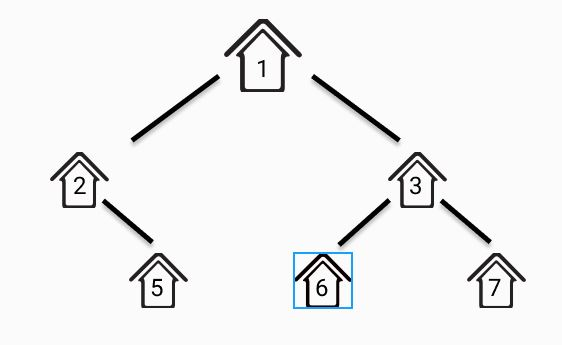# NINJA: The Exam Instructor

Posted: 5 Mar, 2021
Difficulty: Moderate

## PROBLEM STATEMENT

#### For example, consider the following binary tree. The largest subset set is {1, 5, 6, 7} and the size of the subset is 4.##### Input Format:
``````The first line contains an integer 'T' which denotes the number of test cases or queries to be run. Then the 'T' test cases are as follow.

The first line of each test case contains the elements of the tree in the level order form separated by a single space.

If any node does not have a left or right child, take -1 in its place. Refer to the example below.
``````
##### Example:
``````Elements are in the level order form. The input consists of values of nodes separated by a single space in a single line. In case a node is null, we take -1 in its place.

For example, the input for the tree depicted in the below image would be :
````````````1
2 3
4 -1 5 6
-1 7 -1 -1 -1 -1
-1 -1

Explanation :
Level 1 :
The root node of the tree is 1

Level 2 :
Left child of 1 = 2
Right child of 1 = 3

Level 3 :
Left child of 2 = 4
Right child of 2 = null (-1)
Left child of 3 = 5
Right child of 3 = 6

Level 4 :
Left child of 4 = null (-1)
Right child of 4 = 7
Left child of 5 = null (-1)
Right child of 5 = null (-1)
Left child of 6 = null (-1)
Right child of 6 = null (-1)

Level 5 :
Left child of 7 = null (-1)
Right child of 7 = null (-1)

The first not-null node (of the previous level) is treated as the parent of the first two nodes of the current level. The second not-null node (of the previous level) is treated as the parent node for the next two nodes of the current level and so on.

The input ends when all nodes at the last level are null (-1).
``````
##### Note :
``````The above format was just to provide clarity on how the input is formed for a given tree.

The sequence will be put together in a single line separated by a single space. Hence, for the above-depicted tree, the input will be given as:

1 2 3 -1 5 6 7 -1 -1 -1 -1
``````
##### Output Format :
``````For each test case, print the maximum number of elements in a subset satisfying the given condition. Print output of each test case in a separate line.
``````
##### Note:
``````You are not required to print anything explicitly. It has already been taken care of. Just implement the function.
``````
##### Constraints:
``````1 <= T <= 100
1 <= N <= 3000
-10^9 <= DATA <= 10^9

Where ‘T’ is the total number of test cases, ‘N’ is the number of benches(nodes of the tree) in the given class, and 'DATA' is the value mentioned on the bench(value of nodes).

Time Limit: 1sec
``````Approach 1

Approach: We use recursion by using simple two possibilities for every node x, either X is a member of the set or not a member:

• If X is a member, then the value of the maximum subset(X) is 1 plus the maximum subset of all grandchildren.
• If X is not a member, then the value is the sum of the maximum subset of all children.

Here is the algorithm:

1. The logic behind this approach is whether we can choose the child of a node or the grandchild of a node.
2. So for this, we take two variables ‘SIZEEXCL’ and ‘SIZEINCL’, ‘SIZEEXCL’ store the count of a child of the node, and ‘SIZEINCL’ stores the count of the grandchild of the node and recursively we check all the possibility of that node.
3. After checking all the possibilities we return maximum from ‘SIZEINCL’ and ‘SIZEEXCL’.